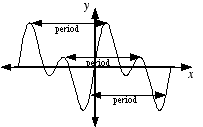index: click on a letter A B C D E F G H I J K L M N O P Q R S T U V W X Y Z A to Z index index: subject areas numbers & symbols sets, logic, proofs geometry algebra trigonometry advanced algebra & pre-calculus calculus advanced topics probability & statistics real world applications multimedia entrieswww.mathwords.com about mathwords website feedback

 Period of a Periodic Function The horizontal distance required for the graph of a periodic function to complete one cycle. Formally, a function f is periodic if there exists a number p such that f(x + p) = f(x) for all x. The smallest possible value of p is the period. The reciprocal of period is frequency.See also